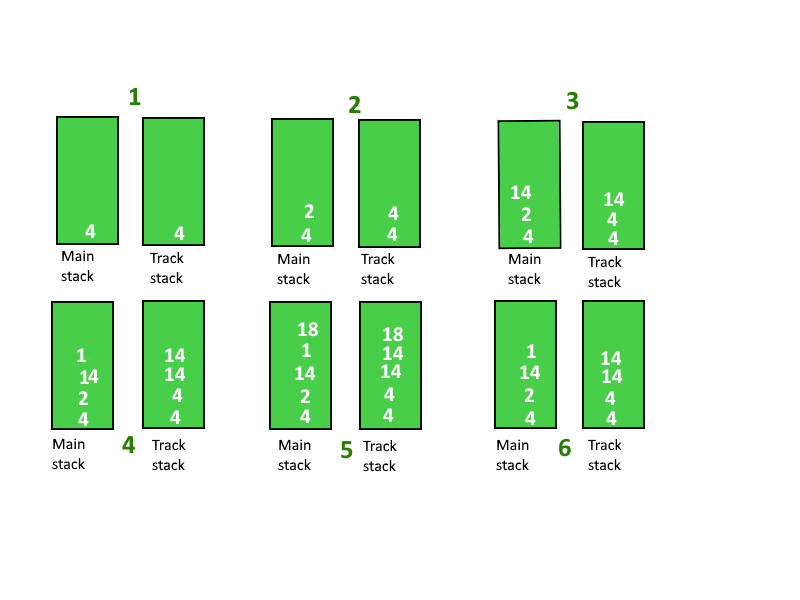# Tracking current Maximum Element in a Stack

• Difficulty Level : Easy
• Last Updated : 19 Jan, 2022

Given a Stack, keep track of the maximum value in it. The maximum value may be the top element of the stack, but once a new element is pushed or an element is popped from the stack, the maximum element will be now from the rest of the elements.
Examples:

```Input : 4 19 7 14 20
Output : Max Values in stack are
4 19 19 19 20

Input : 40 19 7 14 20 5
Output :  Max Values in stack are
40 40 40 40 40 40```

Method 1 (Brute-force): We keep pushing the elements in the main stack and whenever we are asked to return the maximum element, we traverse the stack and print the max element.
Time Complexity : O(n)
Auxiliary Space : O(1)
Method 2 (Efficient): An efficient approach would be to maintain an auxiliary stack while pushing element in the main stack. This auxiliary stack will keep track of the maximum element.
Below is the step by step algorithm to do this

1. Create an auxiliary stack, say ‘trackStack’ to keep the track of maximum element
2. Push the first element to both mainStack and the trackStack.

3. Now from the second element, push the element to the main stack. Compare the element with the top element of the track stack, if the current element is greater than the top of trackStack then push the current element to trackStack otherwise push the top element of trackStack again into it.

4. If we pop an element from the main stack, then pop an element from the trackStack as well.

5. Now to compute the maximum of the main stack at any point, we can simply print the top element of Track stack.

Step by step explanation :
Suppose the elements are pushed on to the stack in the order {4, 2, 14, 1, 18}
Step 1 : Push 4, Current max : 4
Step 2 : Push 2, Current max : 4
Step 3 : Push 14, Current max : 14
Step 4 : Push 1, Current max : 14
Step 5 : Push 18, Current max : 18
Step 6 : Pop 18, Current max : 14Below is the implementation of the above approach:

## C++

 `// C++ program to keep track of maximum``// element in a stack``#include ``using` `namespace` `std;` `class` `StackWithMax``{``    ``// main stack``    ``stack<``int``> mainStack;` `    ``// stack to keep track of max element``    ``stack<``int``> trackStack;` `public``:``    ``void` `push(``int` `x)``    ``{``        ``mainStack.push(x);``        ``if` `(mainStack.size() == 1)``        ``{``            ``trackStack.push(x);``            ``return``;``        ``}` `        ``// If current element is greater than``        ``// the top element of track stack, push``        ``// the current element to track stack``        ``// otherwise push the element at top of``        ``// track stack again into it.``        ``if` `(x > trackStack.top())``            ``trackStack.push(x);``        ``else``            ``trackStack.push(trackStack.top());``    ``}` `    ``int` `getMax()``    ``{``        ``return` `trackStack.top();``    ``}` `    ``int` `pop()``    ``{``        ``mainStack.pop();``        ``trackStack.pop();``    ``}``};` `// Driver program to test above functions``int` `main()``{``    ``StackWithMax s;``    ``s.push(20);``    ``cout << s.getMax() << endl;``    ``s.push(10);``    ``cout << s.getMax() << endl;``    ``s.push(50);``    ``cout << s.getMax() << endl;``    ``return` `0;``}`

## Java

 `// Java program to keep track of maximum``// element in a stack``import` `java.util.*;``class` `GfG {` `static` `class` `StackWithMax``{``    ``// main stack``    ``static` `Stack mainStack = ``new` `Stack ();` `    ``// Stack to keep track of max element``    ``static` `Stack trackStack = ``new` `Stack ();` `static` `void` `push(``int` `x)``    ``{``        ``mainStack.push(x);``        ``if` `(mainStack.size() == ``1``)``        ``{``            ``trackStack.push(x);``            ``return``;``        ``}` `        ``// If current element is greater than``        ``// the top element of track stack, push``        ``// the current element to track stack``        ``// otherwise push the element at top of``        ``// track stack again into it.``        ``if` `(x > trackStack.peek())``            ``trackStack.push(x);``        ``else``            ``trackStack.push(trackStack.peek());``    ``}` `    ``static` `int` `getMax()``    ``{``        ``return` `trackStack.peek();``    ``}` `    ``static` `void` `pop()``    ``{``        ``mainStack.pop();``        ``trackStack.pop();``    ``}``};` `// Driver program to test above functions``public` `static` `void` `main(String[] args)``{``    ``StackWithMax s = ``new` `StackWithMax();``    ``s.push(``20``);``    ``System.out.println(s.getMax());``    ``s.push(``10``);``    ``System.out.println(s.getMax());``    ``s.push(``50``);``    ``System.out.println(s.getMax());``}``}`

## Python3

 `# Python3 program to keep track of``# maximum element in a stack` `class` `StackWithMax:``    ``def` `__init__(``self``):``        ` `        ``# main stack``        ``self``.mainStack ``=` `[]``    ` `        ``# stack to keep track of``        ``# max element``        ``self``.trackStack ``=` `[]` `    ``def` `push(``self``, x):``        ``self``.mainStack.append(x)``        ``if` `(``len``(``self``.mainStack) ``=``=` `1``):``            ``self``.trackStack.append(x)``            ``return` `        ``# If current element is greater than``        ``# the top element of track stack,``        ``# append the current element to track``        ``# stack otherwise append the element``        ``# at top of track stack again into it.``        ``if` `(x > ``self``.trackStack[``-``1``]):``            ``self``.trackStack.append(x)``        ``else``:``            ``self``.trackStack.append(``self``.trackStack[``-``1``])` `    ``def` `getMax(``self``):``        ``return` `self``.trackStack[``-``1``]` `    ``def` `pop(``self``):``        ``self``.mainStack.pop()``        ``self``.trackStack.pop()` `# Driver Code``if` `__name__ ``=``=` `'__main__'``:` `    ``s ``=` `StackWithMax()``    ``s.push(``20``)``    ``print``(s.getMax())``    ``s.push(``10``)``    ``print``(s.getMax())``    ``s.push(``50``)``    ``print``(s.getMax())` `# This code is contributed by PranchalK`

## C#

 `// C# program to keep track of maximum``// element in a stack``using` `System;``using` `System.Collections.Generic;` `class` `GfG``{` `public` `class` `StackWithMax``{``    ``// main stack``    ``static` `Stack<``int``> mainStack = ``new` `Stack<``int``> ();` `    ``// stack to keep track of max element``    ``static` `Stack<``int``> trackStack = ``new` `Stack<``int``> ();` `    ``public` `void` `push(``int` `x)``    ``{``        ``mainStack.Push(x);``        ``if` `(mainStack.Count == 1)``        ``{``            ``trackStack.Push(x);``            ``return``;``        ``}` `        ``// If current element is greater than``        ``// the top element of track stack, push``        ``// the current element to track stack``        ``// otherwise push the element at top of``        ``// track stack again into it.``        ``if` `(x > trackStack.Peek())``            ``trackStack.Push(x);``        ``else``            ``trackStack.Push(trackStack.Peek());``    ``}` `    ``public` `int` `getMax()``    ``{``        ``return` `trackStack.Peek();``    ``}` `    ``public` `void` `pop()``    ``{``        ``mainStack.Pop();``        ``trackStack.Pop();``    ``}``};` `// Driver code``public` `static` `void` `Main()``{``    ``StackWithMax s = ``new` `StackWithMax();``    ``s.push(20);``    ``Console.WriteLine(s.getMax());``    ``s.push(10);``    ``Console.WriteLine(s.getMax());``    ``s.push(50);``    ``Console.WriteLine(s.getMax());``}``}` `/* This code contributed by PrinciRaj1992 */`

## Javascript

 ``

Output:

```20
20
50```

Time Complexity : O(1)
Auxiliary Complexity : O(n)
This article is contributed by Rohit. If you like GeeksforGeeks and would like to contribute, you can also write an article using write.geeksforgeeks.org or mail your article to review-team@geeksforgeeks.org. See your article appearing on the GeeksforGeeks main page and help other Geeks.